# OEImageFrame¶

class OEImageFrame : public OEImageBase


OEImageFrame class provides a convenient way to create a frame with a specific dimension and offset relative to an OEImageBase object. See Figure: Schematic representation of OEImageFrame objects.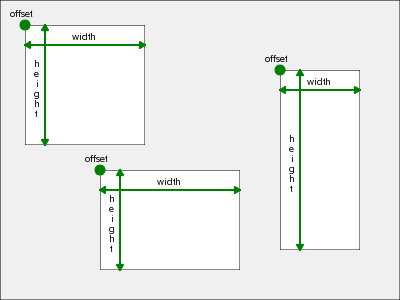Schematic representation of OEImageFrame objects

See also

The following methods are publicly inherited from OEImageBase:

## Constructors¶

OEImageFrame(OEImageBase &parent, double width, double height,
const OE2DPoint &offset)


Creates an OEImageFrame object with the given dimension.

parent
The parent OEImageBase object in which the OEImageFrame object is placed.
width
The width of the OEImageFrame object.
height
The height of the OEImageFrame object.
offset
The offset of the OEImageFrame object relative to top/left corner of its OEImageBase parent.
OEImageFrame(OEImageBase& parent, double padding)


Creates an OEImageFrame object equal paddings around the given image positioning the image frame to the center of the image.

parent
The parent OEImageBase object in which the OEImageFrame object is placed.
padding
The padding that applied in all four sides around the parent image. The padding is capped by the $$\frac{1}{3}$$ of width and height of the parent image.

## Clear¶

void Clear(const OESystem::OEColor &color)


Clears the image with the given color.

See also

## DrawArc¶

void DrawArc(const OE2DPoint &center, double bgnAngle, double endAngle,
double radius, const OEPen &pen)


Draws the outline of an arc. See example in Figure: Example of drawing an arc.

See also

## DrawCircle¶

void DrawCircle(const OE2DPoint &center, double radius, const OEPen &pen)


Draws a circle. See example in Figure: Example of drawing a circle.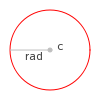Example of drawing a circle

See also

## DrawCubicBezier¶

void DrawCubicBezier(const OE2DPoint &bgn, const OE2DPoint &c1,
const OE2DPoint &c2, const OE2DPoint &end, const OEPen &pen)


Draws a cubic Bézier curve. See example in Figure: Example of drawing a cubic Bezier curve.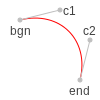Example of drawing a cubic Bezier curve

See also

## DrawLine¶

void DrawLine(const OE2DPoint &bgn, const OE2DPoint &end, const OEPen &pen)


Draws a line. See example in Figure: Example of drawing a line.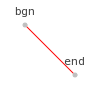Example of drawing a line

See also

## DrawPath¶

void DrawPath(const OE2DPath& path, const OEPen& pen);


Draws a path. See example in Figure: Example of drawing a path.

See also

## DrawPie¶

void DrawPie(const OE2DPoint &center, double bgnAngle, double endAngle,
double radius, const OEPen &pen)


Draws a pie. See example in Figure: Example of drawing a pie.

See also

## DrawPoint¶

void DrawPoint(const OE2DPoint &p, const OESystem::OEColor &color)


Draws a point. See example in Figure: Example of drawing a point.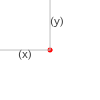Example of drawing a point

See also

## DrawPolygon¶

void DrawPolygon(const std::vector<OE2DPoint> &points, const OEPen &pen)


Draws a closed polygon. See example in Figure: Example of drawing a polygon.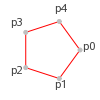Example of drawing a polygon

See also

## DrawQuadraticBezier¶

void DrawQuadraticBezier(const OE2DPoint &bgn, const OE2DPoint &c,
const OE2DPoint &end, const OEPen &pen)


Draws a quadratic Bézier curve. See example in Figure: Example of drawing a quadratic Bezier curve.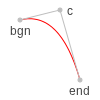Example of drawing a quadratic Bezier curve

See also

## DrawRectangle¶

void DrawRectangle(const OE2DPoint &tl, const OE2DPoint &br, const OEPen &pen)


Draws a rectangle. See example in Figure: Example of drawing a rectangle.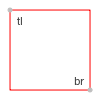Example of drawing a rectangle

See also

## DrawText¶

void DrawText(const OE2DPoint &c, const std::string &text, const OEFont &font,
double maxwidth=0.0)


Draws a text. See example in Figure: Example of drawing a text.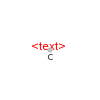Example of drawing a text

See also

## DrawTriangle¶

void DrawTriangle(const OE2DPoint &a, const OE2DPoint &b, const OE2DPoint &c,
const OEPen &pen)


Draws a triangle. See example in Figure: Example of drawing a triangle.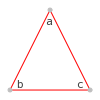Example of drawing a triangle

See also

## GetHeight¶

double GetHeight() const


Returns the height of the OEImageFrame object.

## GetOffset¶

const OE2DPoint &GetOffset() const


Returns the offset of the OEImageFrame object relative to its OEImageBase parent.

## GetWidth¶

double GetWidth() const


Returns the width of the OEImageFrame object.